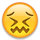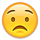# Server Side Processing with PDO/MSSQL

Hi all,

I'm looking to use server side processing to connect to MSSQL, ideally using PDO.

I've looked at the examples included with Datatables but it looks like the ssp.class.php is written specifically for MySQL - Do any examples exist where the functions for searching, filtering, limiting etc are written for MSSQL?

Thanks

Chris

• You are correct - the demo SSP class is specifically for MySQL. I'm not aware of anyone haven't ported it to SQLServer, although given that the latest versions of SQLServer support LIMIT and OFFSET it should be relatively straightforward to do.

Allan

• Thanks Allan,

Unfortunately we aren't using the latest version so it would have to be customised, probably using the row_number() trick i guess - If nobody else has been through it already i'll make a start sometime this week!

Thanks

• please need it right now...

I will share my solution for MSSQL DataTables server side processing just some snip's from my code. Hope it eases some pain.

For awhile, I was tempted to use MariaDB/MySQL just to avoid the hassle encountered working with SQL Server. That solution would, unfortunately, not be acceptable here.

The SSP.PHP is modified from the original to support SQL Server 2014.

PHP on the host

``````\$sql_details = array(
'db'   => 'MY_DATABASE',
'host' => 'MY_HOST,1433'
);
\$table = 'dbo.SomeTableName';
\$primaryKey = 'MYKEYFIELD';
\$columns = array(
array( 'db' => 'col_1', 'dt' => 'A' ),
array( 'db' => 'col_2', 'dt' => 'B' ),
array( 'db' => 'col_3', 'dt' => 'C' ),
array( 'db' => 'col_4', 'dt' => 'D' ),
array( 'db' => 'col_5', 'dt' => 'E' ),
array( 'db' => 'col_6', 'dt' => 'F' ),
array( 'db' => 'col_7', 'dt' => 'G' ),
array( 'db' => 'col_8', 'dt' => 'H' )
);
echo json_encode(SSP::simple( \$_POST, \$sql_details, \$table, \$primaryKey, \$columns ));

// HERE I have appended the modified SSP.PHP source fragments EVERYTHING WORKS
//

/**
* Paging
*/
static function limit ( \$request, \$columns ) {
\$limit = '';
if ( isset(\$request['start']) && \$request['length'] != -1 ) {
\$limit = "ORDER BY [LINE] OFFSET ".intval(\$request['start'])." ROWS FETCH NEXT ".intval(\$request['length'])." ROWS ONLY";
}
// limit and order conflict when using sql server.
// so duplicate the functionality in ORDER and switch on/off as needed based on ORDER
if ( isset(\$request['order'])) {
\$limit = '';    // if there is an ORDER request then clear the limit
return \$limit;    // because the ORDER function will handle the LIMIT
}
else
{
return \$limit;
}
}

/**
* Ordering
*/
static function order ( \$request, \$columns ) {
\$order = '';
if ( isset(\$request['order']) && count(\$request['order']) ) {
\$orderBy = array();
\$dtColumns = self::pluck( \$columns, 'dt' );
for ( \$i=0, \$ien=count(\$request['order']) ; \$i<\$ien ; \$i++ ) {
// Convert the column index into the column data property
\$columnIdx = intval(\$request['order'][\$i]['column']);
\$requestColumn = \$request['columns'][\$columnIdx];
\$columnIdx = array_search( \$requestColumn['data'], \$dtColumns );
\$column = \$columns[ \$columnIdx ];
if ( \$requestColumn['orderable'] == 'true' ) {
\$dir = \$request['order'][\$i]['dir'] === 'asc' ?
'ASC' :
'DESC';
\$orderBy[] = '['.\$column['db'].'] '.\$dir;   // revised for SQL Server
}
}
// see "static function limit" above to explain the next line.
\$order =  "ORDER BY ".implode(', ', \$orderBy)." OFFSET ".intval(\$request['start'])." ROWS FETCH NEXT ".intval(\$request['length'])." ROWS ONLY";
}
return \$order;
}

static function simple ( \$request, \$sql_details, \$table, \$primaryKey, \$columns ) {
\$bindings = array();
\$db = self::sql_connect( \$sql_details );
// Build the SQL query string from the request
\$limit = self::limit( \$request, \$columns );
\$order = self::order( \$request, \$columns );
\$where = self::filter( \$request, \$columns, \$bindings );

// Main query to actually get the data
\$data = self::sql_exec( \$db, \$bindings,
"SET NOCOUNT ON SELECT ".implode(", ", self::pluck(\$columns, 'db'))." FROM \$table \$where \$order \$limit" );

// Data set length after filtering  the \$where will update info OR will be blank when not doing a search
\$resFilterLength = self::sql_exec( \$db, \$bindings,
"SET NOCOUNT ON SELECT ".implode(", ", self::pluck(\$columns, 'db'))." FROM \$table \$where " );
\$recordsFiltered = count(\$resFilterLength);

// Total data set length
\$resTotalLength = self::sql_exec( \$db,"SET NOCOUNT ON SELECT COUNT({\$primaryKey}) FROM \$table" );
\$recordsTotal = \$resTotalLength;

/*  Output   */
return array(
"draw"            => intval( \$request['draw'] ),
"recordsTotal"    => intval( \$recordsTotal ),
"recordsFiltered" => intval( \$recordsFiltered ),
"data"            => self::data_output( \$columns, \$data )
);
}

``````
• Fantastic - thanks for sharing your solution with us.

Allan

• Thanks for Sharing Dan! I'll give it a go.

• Thanks for sharing your solution. I have implemented this with SQL 2012 and it works well. I am using the simple server side example just to get started. I had to also modify the SQL connect funnction in the ssp.class.php file as well (see below).

The one thing that still doesn't seem to work is the search functionality. When I even type one letter into the search, I immediately get an error that says

DataTables warning: table id=example - An SQL error occurred: SQLSTATE: [Microsoft][SQL Server Native Client 11.0][SQL Server]Incorrect syntax near '`'.

Any idea how to get around this issue?

``````static function sql_connect ( \$sql_details )
{
try {
\$db = @new PDO(
"sqlsrv:server={\$sql_details['host']};database={\$sql_details['db']}",
\$sql_details['user'],
\$sql_details['pass'],
array( PDO::ATTR_ERRMODE => PDO::ERRMODE_EXCEPTION )
);
}
catch (PDOException \$e) {
self::fatal(
"An error occurred while connecting to the database. ".
"The error reported by the server was: ".\$e->getMessage()
);
}

return \$db;
}
``````
• SQL Server doesn't use '`' as a field escape character like MySQL does. You would need to go through the SSP class and update the SQL that is generated to be suitable for SQL Server.

Allan

• Thanks Allan ! I responded privately to pastorjustin thinking I broke something in the SQLserver coding myself.

• Apologies for reviving an old thread but this thread, in particular DanF's code, has helped me out massively with SSP on SQL server, I don't think I'd have got it working without that code. Switching to MySQL was out of the question because it was a work project.

One thing I did find was the queries for getting total data set length and filtered data set length slowed the table down a lot, and, being SSP, meant that every page turn, filter and sort would have this delay (12 seconds roughly). I fixed that issue by using SELECT COUNT({\$primaryKey}), which brought the times down to around 2 seconds. I've also been using ssp:complex rather than ssp:simple which DanF had modified for SQL Server above, so here is my code for that:

``````    static function complex ( \$request, \$conn, \$table, \$primaryKey, \$columns, \$whereResult=null, \$whereAll)
{
\$bindings = array();
\$db = self::db( \$conn );
\$localWhereResult = array();
\$localWhereAll = array();
\$whereAllSql = '';

// Build the SQL query string from the request
\$limit = self::limit( \$request, \$columns );
\$order = self::order( \$request, \$columns );
\$where = self::filter( \$request, \$columns, \$bindings );

\$whereResult = self::_flatten( \$whereResult );
\$whereAll = self::_flatten( \$whereAll );

if ( \$whereResult ) {
\$where = \$where ?
\$where .' AND '.\$whereResult :
'WHERE '.\$whereResult;
}

if ( \$whereAll ) {
\$where = \$where ?
\$where .' AND '.\$whereAll :
'WHERE '.\$whereAll;

//\$whereAllSql = 'WHERE '.\$whereAll;
}

// Main query to actually get the data
\$data = self::sql_exec( \$db, \$bindings,
//        "SET NOCOUNT ON SELECT ".implode(", ", self::pluck(\$columns, 'db'))." FROM \$table \$where \$order \$limit" );
"SET NOCOUNT ON SELECT * FROM \$table \$where \$order \$limit" );

// Data set length after filtering
\$resFilterLength = self::sql_exec( \$db, \$bindings,
"SELECT count({\$primaryKey}) FROM \$table \$where" );
\$recordsFiltered = \$resFilterLength;

// Total data set length
\$resTotalLength = self::sql_exec( \$db,"SELECT COUNT({\$primaryKey}) FROM \$table" );
\$recordsTotal = \$resTotalLength;

/*
* Output
*/
return array(
"draw"            => isset ( \$request['draw'] ) ?
intval( \$request['draw'] ) :
0,
"recordsTotal"    => \$recordsTotal,
"recordsFiltered" => \$recordsFiltered,
"data"            => self::data_output( \$columns, \$data )
);
}
``````

I might have revived this thread for nothing but hopefully my contribution will help somebody out there.

Another thing to note is that this won't work if your version of SQL Server doesn't support LIMIT and OFFSET, anything later than 2008 should work (and possibly 2008 with service packs, not entirely sure on that). Just do a simple query with LIMIT and OFFSET to check if it works first.

• This is excellent. Thank you for providing a working version for SQL Server 2012+ but I hope there is a working version for anything prior to 2012 without LIMIT and OFFSET. I'm in a dilemma as my working environment is reluctant to upgrade to 2012 from 2008. If anyone has it, PLEASE contribute!• This SO thread discusses how SQL Server 2008 can do an OFFSET / LIMIT type query. You'd need to modify the above script to use that nesting query.

Allan

• I've had to do a table with 2008 before, I didn't use DataTables, although I could have if I knew then what I know now about SSP in DataTables.

If you're SQL is quite good (or you have a colleague you can throw it at) then you could develop a stored procedure using the form data sent by DataTables to the PHP file. For something simple you'd only need to pass the stored procedure the page length and start row, then if you wanted sorting you'd need to give it the sort column and sort direction, for filters the column to be filtered and the filter value. You'd need to give each row a row number after the filtering and sorting, then select row number between start row and (start row + page length). Pass the data back in a JSON object which DataTables can understand.

I may have made that sound easier than it actually is, but it's how I'd do it now if I had to use 2008.

• IanRCarter, what alternative script did you use back then with 2008? I'm pretty much exhausted and can't figure out how to mod the SSP query correctly--even with Alan's great suggestion. After a week and a half of trial-and-error, I may throw in the towel.This discussion has been closed.